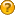###Author Topic: How to calculate area?  (Read 2370 times)

####Alexei_B

• byte
•• Posts: 4##### How to calculate area?
« on: December 13, 2007, 05:14:40 pm »
I've parked my attempt to calculate volume for now, and I'm concentrating on simple areas of polygons instead.

My method is to call (pseudo code)

polyManager.getTransformedVertex(polygon,vertex1)
polyManager.getTransformedVertex(polygon,vertex2)
polyManager.getTransformedVertex(polygon,vertex3)

on a polygon. This gives me three vertices which I can then put into a general formula for triangle areas.

However, because these are Transformed verices, i.e. in World Space, the calculated area changes as a object is rotated. I need the absolute vertices of the underlying mesh, so I thought to apply

Matrix mtTransform =obj.getWorldTransformation().invert();

to "untransform" from World to Object vertices.

Does this rather clumsy method sound appropriate?  Any comments welcome.

####raft

••• Posts: 1986##### Re: How to calculate area?
« Reply #1 on: December 13, 2007, 07:44:33 pm »
why should you transform vertices back to original position ? the area doesnt change as the polygons arent distorted (like sheer etc)

r a f t

####Alexei_B

• byte
•• Posts: 4##### Re: How to calculate area?
« Reply #2 on: December 14, 2007, 11:03:26 am »
I can see why you ask that question.

For some reason, as I rotate my simple cube, the area calculation changes for a given triangle on the face of the cube.  This seems to be because of the rotation - the visible area decreases as the selected triangle turns away from the view point.

It is because of this that I was concerned about the getTransformedVertex call, and was seeking to "untransform" it.

Perhaps I should be trying to calculate area on the underlying mesh?

####raft

••• Posts: 1986##### Re: How to calculate area?
« Reply #3 on: December 14, 2007, 03:03:34 pm »
this shouldnt happen. as the cube turns only the area of projection of polygons change, for example projection to screen, the part we see. the area of polygons shouldnt change

maybe there is a flaw in your are calculations ?

####Alexei_B

• byte
•• Posts: 4##### Re: How to calculate area?
« Reply #4 on: December 14, 2007, 04:04:30 pm »
Hi raft,

You are absolutely right.  I've changed the way the area is calculated, and now use a vector cross product, i.e

area = | a x b |  / 2

which gives consistent results despite orientation of the cube.

Thanks for your help raft, and sorry for troubling the forum about what is my mistake.